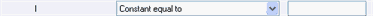## Please enable JavaScript to view this site.

 Entering default constraints

## What is a constraint?

Constraining parameters is often essential to getting useful results. Constraints can be used to fix parameters to constant values, to share parameters among data sets (global fitting), and to define one parameter to be a column constant (whose value comes from the column titles in the data table).

## Defining constraints when defining an equation

You can define default constraints as part of the equation definition. This is useful for constraints that will apply every time the equation is used.

Constraints can be for one parameter (Kfast must be greater than zero) or for the relationship between two parameters (Kfast must be greater than Kslow). But note that you cannot invoke a constraint between two parameters if both parameters also are themselves constrained. In a two phase exponential equation, you may want to constrain both parameters to be greater than zero, and also define one rate constant to be larger than the other (Kfast > Kslow). Prism won't let you do that. What you have to do is define one constraint that Kfast is greater than zero, and another that Kfast is greater than Kslow. But don't put in the constraint that Kslow is greater than zero. That is implied by being larger than Kfast.

## Defining experimental constants

In some cases, one of the parameters in the equation is an experimental constant. It needs to be set to a constant value, but that value differs each time you run the experiment. Do that by going to the constraints tab of the nonlinear regression dialog every time that equation is used.

When you define the equation, you can add a constraint that reminds anyone using the equation to set that constant value. In the constraints tab when defining the equation, choose the constraint "Constant equal to" but leave the value (to the right) blank. With an equation defined this way, Prism won't fit data to that equation until the parameter is defined (or the constraint changed).